# Post-processing quantities: translation motion)

## Introduction

This paragraph deals with physical quantities computed for translating motion.

## Kinematic variables

The list of kinematic variables used for solving and the names of the corresponding Flux parameters are given in the table below; in this table MS indicates the concerned mechanical set.

Kinematic variable Name Unit
Linear position: y LinPos(MS) m
Linear speed: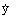LinSpeed(MS) m/s
Linear acceleration:LinAcc(MS) m/s²

## Physical quantities

The list of physical quantities computed in the post-processing module and the names of the corresponding Flux parameters are given in the table below; in this table MS indicates the concerned mechanical set.

Force Name Unit Explanation
Electromagnetic force: F em ForceElecMag(MS) N
Internal system
Friction force: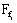ForceFrInt(MS) N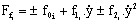Spring return force: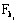ForceSpInt(MS) N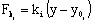Inertia force: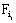ForceInInt(MS) N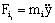Resistant force*: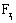ForceReInt(MS) N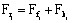or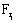External system
Friction force: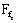ForceFrExt(MS) N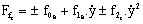Spring return force: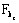ForceSpExt(MS) N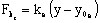Inertia force: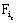ForceInExt(MS) N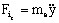Resistant force * :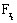ForceReExt(MS) N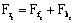or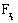Note: *The resistant force is:
• either the sum of the friction force + the spring return force
• or the resistant force defined using a formula

## Reminder of the solved mechanical equation

The solved mechanical equation for translating motion is: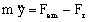where:

• m is the mass•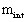is the mass of the moving mechanical set (internal system)
•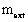is the mass of the external load (external system)
•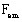is the electromagnetic force
•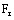is the resistant force•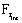is the resistant force acting on the moving mechanical set (internal system)
•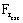is the resistant force acting on the external load (external system)

## Important note

When there are symmetries and periodicities, the forces are computed for the entire device.